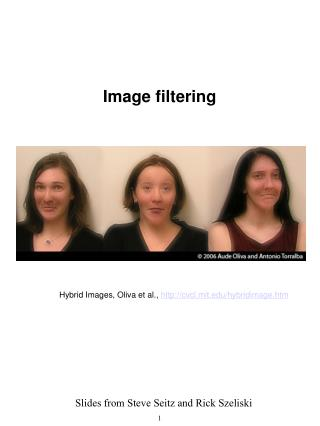DownloadDownload PresentationImage filtering

# Image filtering

Download Presentation## Image filtering

- - - - - - - - - - - - - - - - - - - - - - - - - - - E N D - - - - - - - - - - - - - - - - - - - - - - - - - - -
##### Presentation Transcript

1. Image filtering Hybrid Images, Oliva et al., http://cvcl.mit.edu/hybridimage.htm Slides from Steve Seitz and Rick Szeliski

2. Image filtering Hybrid Images, Oliva et al., http://cvcl.mit.edu/hybridimage.htm

3. What is an image?

4. Images as functions • We can think of an image as a function, f, from R2 to R: • f( x, y ) gives the intensity at position ( x, y ) • Realistically, we expect the image only to be defined over a rectangle, with a finite range: • f: [a,b]x[c,d]  [0,1] • A color image is just three functions pasted together. We can write this as a “vector-valued” function:

5. Images as functions

6. What is a digital image? • We usually work with digital (discrete)images: • Sample the 2D space on a regular grid • Quantize each sample (round to nearest integer) • If our samples are D apart, we can write this as: • f[i ,j] = Quantize{ f(iD, jD) } • The image can now be represented as a matrix of integer values

7. Filtering noise • How can we “smooth” away noise in an image?

8. Mean filtering

9. Mean filtering

10. We can generalize this idea by allowing different weights for different neighboring pixels: This is called a cross-correlation operation and written: H is called the “filter,” “kernel,” or “mask.” The above allows negative filter indices. When you implement need to use: H[u+k,v+k] instead of H[u,v] Cross-correlation filtering • Let’s write this down as an equation. Assume the averaging window is (2k+1)x(2k+1):

11. Mean kernel • What’s the kernel for a 3x3 mean filter?

12. Mean vs. Gaussian filtering

13. Gaussian filtering • A Gaussian kernel gives less weight to pixels further from the center of the window • This kernel is an approximation of a Gaussian function: • What happens if you increase  ?Photoshop demo

14. Image gradient • How can we differentiate a digital image F[x,y]? • Option 1: reconstruct a continuous image, f, then take gradient • Option 2: take discrete derivative (finite difference) How would you implement this as a cross-correlation?

15. Image gradient It points in the direction of most rapid change in intensity

16. The gradient direction is given by: • how does this relate to the direction of the edge? • The edge strength is given by the gradient magnitude Image gradient It points in the direction of most rapid change in intensity

17. original smoothed (5x5 Gaussian) Why doesthis work? smoothed – original (scaled by 4, offset +128)

18. Gaussian delta function Laplacian of Gaussian (2nd derivative operator)

19. Convolution • A convolution operation is a cross-correlation where the filter is flipped both horizontally and vertically before being applied to the image: • It is written: • Suppose H is a Gaussian or mean kernel. How does convolution differ from cross-correlation? • Suppose F is an impulse function (previous slide) What will G look like?

20. Continuous Filters • We can also apply filters to continuous images. • In the case of cross correlation: • In the case of convolution: • Note that the image and filter are infinite.

21. More on filters… • Cross-correlation/convolution is useful for, e.g., • Blurring • Sharpening • Edge Detection • Interpolation • Convolution has a number of nice properties • Commutative, associative • Convolution corresponds to product in the Fourier domain • More sophisticated filtering techniques can often yield superior results for these and other tasks: • Polynomial (e.g., bicubic) filters • Steerable filters • Median filters • Bilateral Filters • … • (see text, web for more details on these)